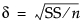Raw effect size. For details about effect size computations, see Effect Size in the Fitting Linear Models book. The first position is initially set to the square root of the sums of squares for the hypothesis divided by the square root of n; that is,.
Solves for the value of the parameter or linear test that produces a p-value of α. This is a function of α, σ, n, and the standard error of the estimate. This feature is available only when the X factor has two levels and is usually used for individual parameters.
 – Adjusted power is the power calculated from a more unbiased estimate of the non-centrality parameter.
 – The confidence interval for the adjusted power is based on the confidence interval for the non-centrality estimate.
Adjusted power and confidence limits are computed only for the original Delta, because that is where the random variation is.
Related Information
 • Example of the Power Option
 • Power

Help created on 9/19/2017